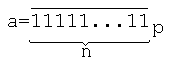시간 제한메모리 제한제출정답맞힌 사람정답 비율
1 초 128 MB111100.000%

## 문제The form of a natural number a in a number system with base p contains of n ones (digts "1") in a row.

Your task is to write a program that computes the greatest values of exponents of numbers 2 and 3 such that the remainder of a divided by both these values the remainder is 0.

## 입력

Two values of natural numbers p (1 < p < 109) and n (n < 109) are input from the keyboard.

## 출력

Two non-negative integer numbers must be output on the screen: the values of the greatest exponents of two and three.

## 예제 입력 1

17 2


## 예제 출력 1

1 2


## 예제 입력 2

10 4


## 예제 출력 2

0 0